International
Tables for
Crystallography
Volume A
Space-group symmetry
Edited by Th. Hahn

International Tables for Crystallography (2006). Vol. A, ch. 2.2, pp. 18-19

## Section 2.2.4.1. Present symbols

Th. Hahna* and A. Looijenga-Vosb

aInstitut für Kristallographie, Rheinisch-Westfälische Technische Hochschule, Aachen, Germany, and bLaboratorium voor Chemische Fysica, Rijksuniversiteit Groningen, The Netherlands
Correspondence e-mail:  hahn@xtl.rwth-aachen.de

#### 2.2.4.1. Present symbols

| top | pdf |

Both the short and the full Hermann–Mauguin symbols consist of two parts: (i) a letter indicating the centring type of the conventional cell, and (ii) a set of characters indicating symmetry elements of the space group (modified point-group symbol).

• (i) The letters for the centring types of cells are listed in Chapter 1.2. Lower-case letters are used for two dimensions (nets), capital letters for three dimensions (lattices).

• (ii) The one, two or three entries after the centring letter refer to the one, two or three kinds of symmetry directions of the lattice belonging to the space group. These symmetry directions were called blickrichtungen by Heesch (1929). Symmetry directions occur either as singular directions (as in the monoclinic and orthorhombic crystal systems) or as sets of symmetrically equivalent symmetry directions (as in the higher-symmetrical crystal systems). Only one representative of each set is required. The (sets of) symmetry directions and their sequence for the different lattices are summarized in Table 2.2.4.1. According to their position in this sequence, the symmetry directions are referred to as primary', secondary' and tertiary' directions.

 Table 2.2.4.1| top | pdf | Lattice symmetry directions for two and three dimensions
 Directions that belong to the same set of equivalent symmetry directions are collected between braces. The first entry in each set is taken as the representative of that set.
LatticeSymmetry direction (position in Hermann–Mauguin symbol)
PrimarySecondaryTertiary
Two dimensions
Oblique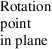Rectangular  
Square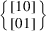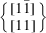Hexagonal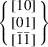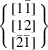Three dimensions
Triclinic None
Monoclinic  (unique axis b')
 (unique axis c')
Orthorhombic   
Tetragonal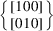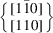Hexagonal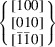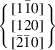Rhombohedral (hexagonal axes)Rhombohedral (rhombohedral axes)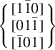Cubic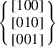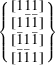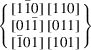For the full Hermann–Mauguin symbols see Section 2.2.4.1.

This sequence of lattice symmetry directions is transferred to the sequence of positions in the corresponding Hermann–Mauguin space-group symbols. Each position contains one or two characters designating symmetry elements (axes and planes) of the space group (cf. Chapter 1.3) that occur for the corresponding lattice symmetry direction. Symmetry planes are represented by their normals; if a symmetry axis and a normal to a symmetry plane are parallel, the two characters (symmetry symbols) are separated by a slash, as in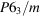or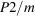(two over m').

For the different crystal lattices, the Hermann–Mauguin space-group symbols have the following form:

 (i) Triclinic lattices have no symmetry direction because they have, in addition to translations, only centres of symmetry,. Thus, only two triclinic space groups, P1 (1) and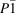(2), exist. (ii) Monoclinic lattices have one symmetry direction. Thus, for monoclinic space groups, only one position after the centring letter is needed. This is used in the short Hermann–Mauguin symbols, as in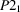. Conventionally, the symmetry direction is labelled either b (unique axis b') or c (unique axis c'). In order to distinguish between the different settings, the full Hermann–Mauguin symbol contains two extra entries 1'. They indicate those two axial directions that are not symmetry directions of the lattice. Thus, the symbols P121, P112 and P211 show that the b axis, c axis and a axis, respectively, is the unique axis. Similar considerations apply to the three rectangular plane groups pm, pg and cm (e.g. plane group No. 5: short symbol cm, full symbol c1m1 or c11m). (iii) Rhombohedral lattices have two kinds of symmetry directions. Thus, the symbols of the seven rhombohedral space groups contain only two entries after the letter R, as in R3m or R3c. (iv) Orthorhombic, tetragonal, hexagonal and cubic lattices have three kinds of symmetry directions. Hence, the corresponding space-group symbols have three entries after the centring letter, as in Pmna, P3m1, P6cc or. Lattice symmetry directions that carry no symmetry elements for the space group under consideration are represented by the symbol 1', as in P3m1 and P31m. If no misinterpretation is possible, entries 1' at the end of a space-group symbol are omitted, as in P6 (instead of P611),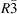(instead of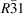),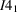(instead of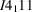), F23 (instead of F231); similarly for the plane groups.

Short and full Hermann–Mauguin symbols differ only for the plane groups of class m, for the monoclinic space groups, and for the space groups of crystal classes mmm,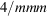,,,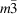and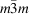. In the full symbols, symmetry axes and symmetry planes for each symmetry direction are listed; in the short symbols, symmetry axes are suppressed as much as possible. Thus, for space group No. 62, the full symbol is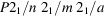and the short symbol is Pnma. For No. 194, the full symbol is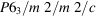and the short symbol is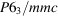. For No. 230, the two symbols are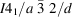and.

Many space groups contain more kinds of symmetry elements than are indicated in the full symbol (additional symmetry elements', cf. Chapter 4.1). A complete listing of the symmetry elements is given in Tables 4.2.1.1and 4.3.2.1under the heading Extended full symbols. Note that a centre of symmetry is never explicitly indicated (except for space group); its presence or absence, however, can be readily inferred from the space-group symbol.

### References

Heesch, H. (1929). Zur systematischen Strukturtheorie. II. Z. Kristallogr. 72, 177–201.Google Scholar